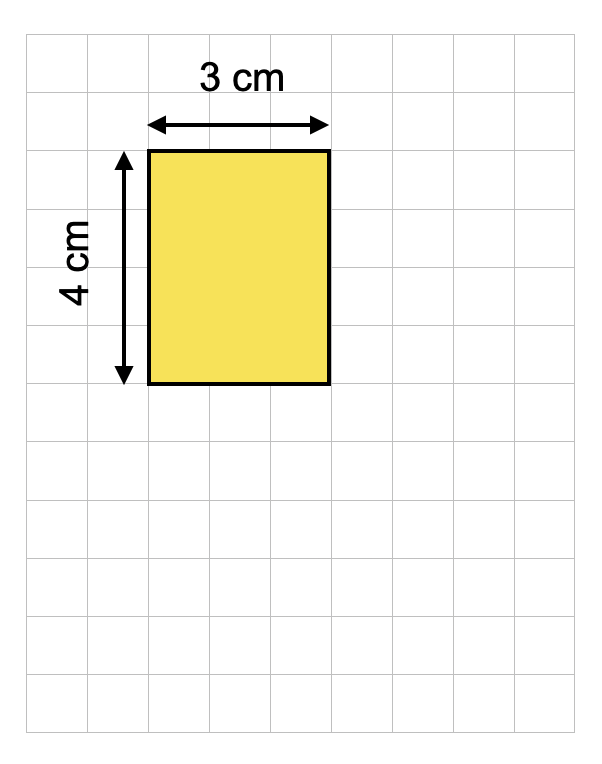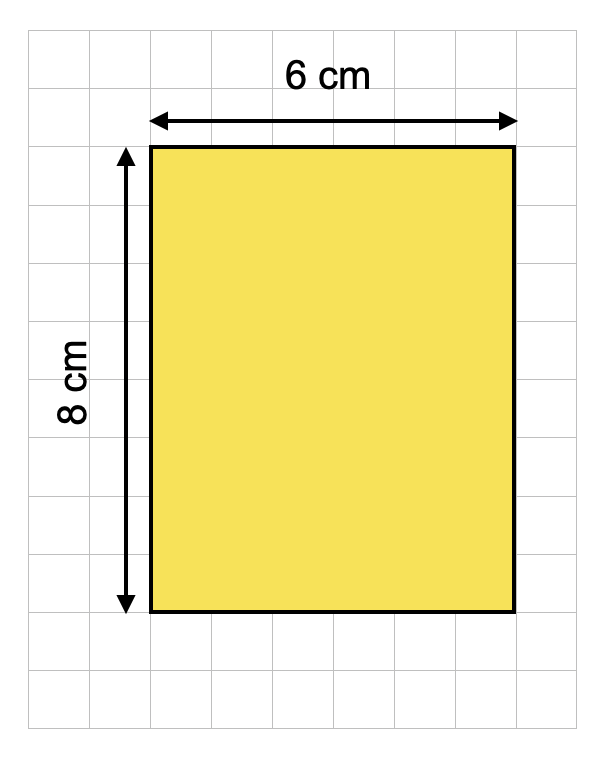# Scaling up

## What is scaling?

Scaling is a method of increasing or reducing the size of an object or measurement using the information you have about it.

## Scaling up

Scaling up means making something larger, or increasing its size.

In order to do this, you first have to know the original measurements of the object or objects that you are scaling up, and then use your knowledge of multiplication.

Take a look at this rectangle. The shape on the right has been scaled up to twice the size of the shape on the left:The length and width measurements of the rectangle have both been multiplied by 2 in order to make the shape twice its original size:

2 x 3 cm = 6 cm

2 x 4 cm = 8 cm

We can also scale up amounts. Take a look at the ingredients for this recipe:

Chocolate Chip Muffins (makes 10)

2 eggs

200g flour

120g sugar

100g butter

50g chocolate chips

If we wanted to make 30 muffins for a party (three times more than the amount the original recipe makes), then we would need to scale up the ingredients by multiplying each amount by 3:

3 x 2 = 6 eggs

3 x 200g = 600g flour

3 x 120g = 360g sugar

3 x 100g = 300g butter

3 x 50g = 150g chocolate chips

## Scaling down

Scaling down means making something smaller, or reducing its size.

In order to do this, you first have to know the original measurements of the object or objects that you are scaling up, and then use your knowledge of division.

Take a look at this rectangle. The shape on the right has been scaled down so it is three times smaller than the shape on the left:

The length and width measurements of the rectangle have both been divided by 3 in order to make the shape three times smaller than its original size:

6 cm ÷ 3 = 2 cm

3 cm ÷ 3 = 1 cm

We can also scale down amounts. Take a look at the ingredients for this recipe:

300g plain flour

240g butter

200g sugar

120g smarties

2 eggs

2 tsp baking powder

If we wanted to make just 5 cookies (four times less than the amount the original recipe makes), then we would need to scale down the ingredients by dividing each amount by 4:

300g ÷ 4 = 75g plain flour

240g ÷ 4 = 60g butter

200g ÷ 4 = 50g sugar

120g ÷ 4 = 40g smarties

2 eggs ÷ 4 = 1/2 egg

2 tsp ÷ 4 = 1/2 tsp baking powder

## Scale factor

The number that we multiply a measurement by is called the scale factor.

We can work out what the scale factor from square A to square B is by looking at the relationship between the two measurements:

We need to calculate what number 5 is multiplied to get an answer of 15:

5 x 3 = 15

So, the scale factor is 3.

## Scale drawings

A scale drawing is a drawing that shows a real object with the measurements reduced or increased by a scale factor.

Maps are a real-life example of scale drawings. A map scale refers to the relationship between distance on a map and the corresponding distance on the ground. For example, on a 1:25,000 scale map, 1cm on the map equals 25,000cm on the ground.

Architects, engineers and designers also produce scale drawings of the buildings or objects that they are designing.

## Scaling KS2

The concept of scaling appears in the National Curriculum at KS2, in the Number - multiplication and division section for Year 3, 4, and 5, and the Ratio and Proportion section for Year 6:

## Scaling Year 3

• solve problems, including missing number problems, involving multiplication and division, including positive integer scaling problems and correspondence problems in which n objects are connected to m objects.

## Scaling Year 4

• solve problems involving multiplying and adding, including using the distributive law to multiply two digit numbers by one digit, integer scaling problems and harder correspondence problems such as n objects are connected to m objects.

## Scaling Year 5

• solve problems involving multiplication and division, including scaling by simple fractions and problems involving simple rates.

## Scaling Year 6

• solve problems involving similar shapes where the scale factor is known or can be found